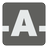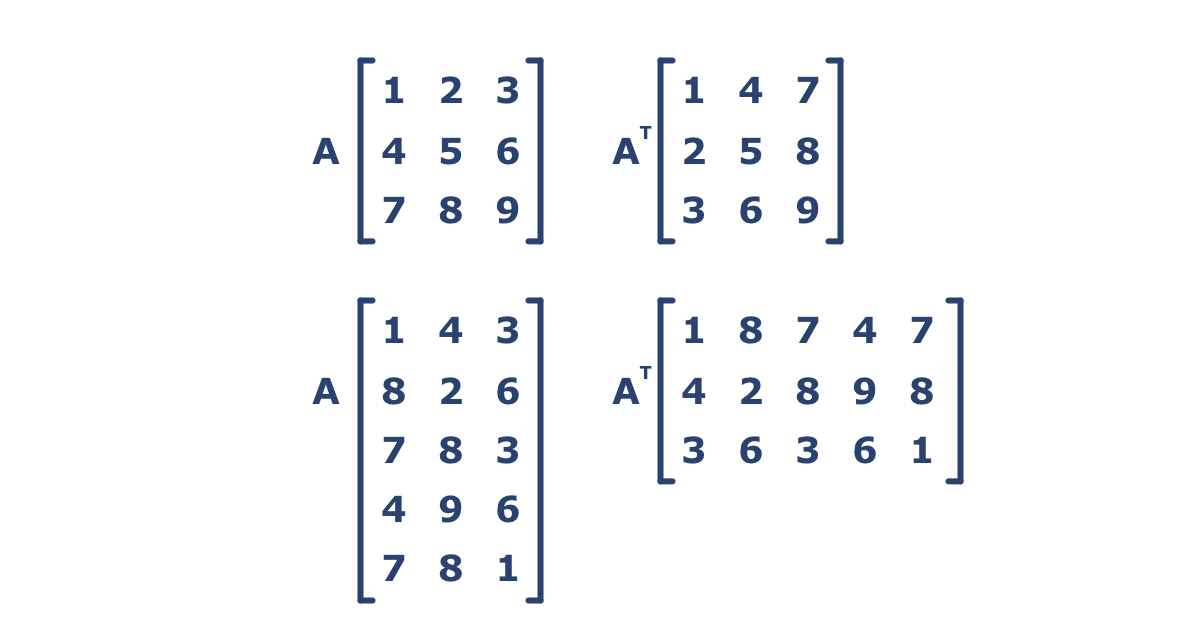Transposed Matrix
Simple
English FR RU

In linear algebra, the transpose of a matrix A is another matrix AT (also written A′, Atr,tA or At) created by any one of the following equivalent actions:

• reflect A over its main diagonal (which runs from top-left to bottom-right) to obtain AT
• write the rows of A as the columns of AT
• write the columns of A as the rows of AT

Formally, the ith row, jth column element of AT is the jth row, ith column element of A:

[AT]i j = [A]j i

If A is an m × n matrix then AT is an n × m matrix.

You have been given a matrix as a 2D list with integers. Your task is to return a transposed matrix based on input.Input: A matrix as a list of lists with integers.

Output: The transposed matrix as a list/tuple of lists/tuples with integers.

Example:

```checkio([[1, 2, 3],
[4, 5, 6],
[7, 8, 9]])...```
You should be an authorized user in order to see the full description and start solving this mission.